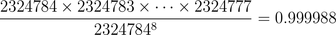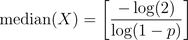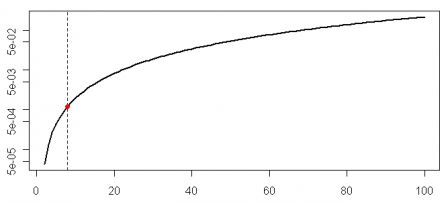November 2, 2010
By

Want to share your content on R-bloggers? click here if you have a blog, or here if you don't.

The only thing I remember from courses I had in probability a
few years ago is that we also have to clearly defined the
event we want to calculate the probability. On the Freakonomics blog,
where I mentioned that, and odds facts from the French lottery),Yesterday, Andrew Gelman claimed (here)
that there was a probability
error
… Well, since Andrew is really a statistician (and a good one… while I am
barely an economist), I tried to do the maths….
and to understand where the error was coming from…
Since 6 numbers are drawn out of a pool of numbers from 1 to 37, the total
number of combination at each lottery is> (n=choose(37,6))
 2324784

Over 8 lotteries (since there are two draws per week, we can assume there 8 draws
per month)
, the probability of no identical
draws isHere is the R code for those who want to check, again,
> prod(n-0:7)/n^8
 0.999988

Each month, the probability of “coincidence” (I define “coincidence” the
event “over 8 draws, at
least two times, we obtained the same 6-uplet
” or more precisely (as mentioned here)
over one calendar month, at
least two times, we obtained the same 6-uplet
) is
p=1.204407e-05.
> (p=1-(prod(n-0:7)/n^8))
 1.204407e-05

The occurrence of a coincidence each month as a Geometric distribution,
with probability p. And it is classical, following Gumbel’s definition (here),
to consider 1/p, called the “return
period
“, i.e. the number of months we have to wait until
we observe a coincidence (i.e. a repetition in the same month), since
for a geometric distribution>
1/p/(12)
 6919.034

Here, the (expected) return period is 6919 years.
From my point of view, this is “the incident of six numbers
repeating themselves within a calendar month
”, and this is an event of
once in 6919.034 years. On the other hand the median of a geometric
distribution is> -log(2)/log(1-p)/(12)
 4795.88

which means that we have 50%
chance to get such a coincidence over 4796 years.

looking at a longer period, say 100 draws, i.e. one year
(here
I define “coincidence
the event “over 100
draws, at least two times, we obtained the same 6-uplet
“),
we have in red the expected return period, and in blue the median of the geometric distribution,> M=E=rep(NA,100)
> for(i in 2:100){
+ p=1-exp((sum(log(n-0:(i-1)))-i*log(n)))
+ E[i]=1/p/(100/i)
+ M[i]=-log(2)/log(1-p)/(100/i)
+ }
> plot(1:100,E,ylim=c(0,10000),type=”l”,col=”red”,lwd=2)
> lines(1:100,M,col=”blue”,lwd=2)
> abline(v=8,lty=2)
> points(8,E,pch=19,col=”red”)
> points(8,M,pch=19,col=”blue”)

or below of a log-scaled versionAs Xi’an did (here), assume now that there is a lottery over 100
countries. Here I define “coincidence
the event “over k
lottery draws over 100 around the world, at least two times, we
obtained the same 6-uplet
“,
and then the previous graph becomes (with on the x axis the level of k)Here I have a 12% chance if we consider probability to have identical numbers over a month…
But here, we can have one 6-uplet in Israel, and the other one in Egypt, say… If we want to get the same 6-uplet in the same country, the graph is nowi.e. each month there is a chance over one thousand…
> i=8
> p=1-exp((sum(log(n-0:(i-1)))-i*log(n)))
> 1-(1-p)^100
 0.001203689

Note:
actually, Xi’an mentioned that the probability that this coincidence [of
two identical draws over 188 draws] occurred in at least one out of 100
lotteries (there are hundreds of similar lotteries across the World) is
53%! And I got the same,
> 1-(1-P)^100
 0.5305219

R-bloggers.com offers daily e-mail updates about R news and tutorials about learning R and many other topics. Click here if you're looking to post or find an R/data-science job.
Want to share your content on R-bloggers? click here if you have a blog, or here if you don't.

Tags: , , , , ,